## 使用Python进行音频处理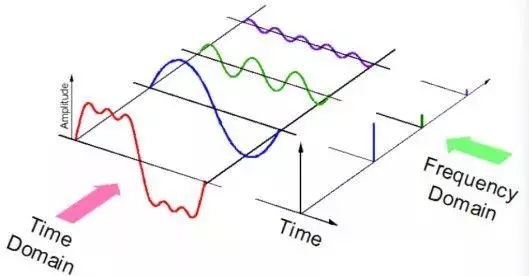• mp3格式
• wma（Windows Media Audio）格式
• wav（波形音频文件）格式

### 音频库

Python有一些很棒的音频处理库，比如Librosa和PyAudio。还有一些内置的模块用于一些基本的音频功能。

#### 安装

pip install librosa
# or
conda install -c conda-forge librosa


#### 2. IPython.display.Audio

IPython.display.Audio 让您直接在jupyter笔记本中播放音频。

import librosa

audio_path = '../T08-violin.wav'
x , sr = librosa.load(audio_path)
print(type(x), type(sr))
# <class 'numpy.ndarray'> <class 'int'>
print(x.shape, sr)
# (396688,) 22050


librosa.load(audio_path, sr=44100)


librosa.load(audio_path, sr=None)


#### 播放音频

import IPython.display as ipd
ipd.Audio(audio_path)### 可视化音频

##### 波形

import matplotlib.pyplot as plt
import librosa.display

plt.figure(figsize=(14, 5))
librosa.display.waveplot(x, sr=sr)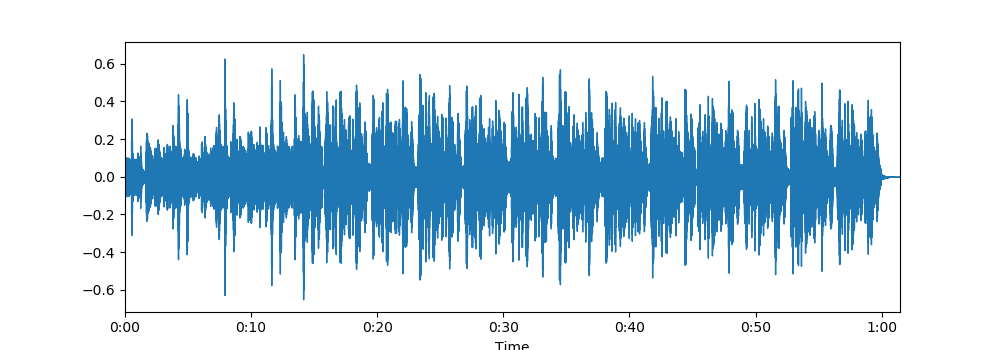##### 声谱图(spectrogram)

X = librosa.stft(x)
Xdb = librosa.amplitude_to_db(abs(X))
plt.figure(figsize=(14, 5))
librosa.display.specshow(Xdb, sr=sr, x_axis='time', y_axis='hz')
plt.colorbar()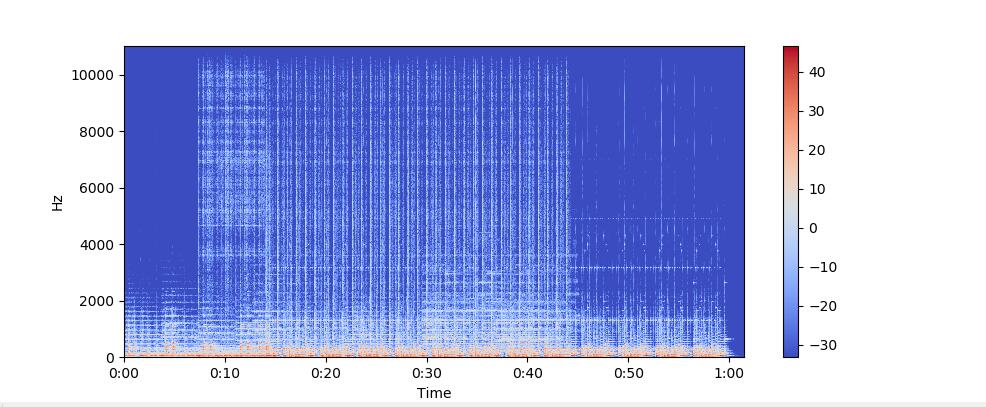librosa.display.specshow(Xdb, sr=sr, x_axis='time', y_axis='log')
plt.colorbar()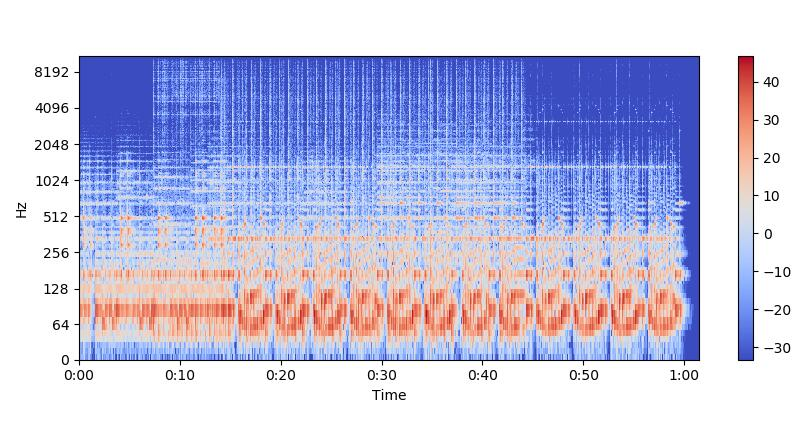##### 写音频

librosa.output.write_wav 将NumPy数组保存到WAV文件。

librosa.output.write_wav('example.wav', x, sr)


### 创建音频信号

import numpy as np
sr = 22050 # sample rate
T = 5.0    # seconds
t = np.linspace(0, T, int(T*sr), endpoint=False) # time variable
x = 0.5*np.sin(2*np.pi*220*t)# pure sine wave at 220 Hz
Playing the audio
ipd.Audio(x, rate=sr) # load a NumPy array
Saving the audio
librosa.output.write_wav('tone_220.wav', x, sr)


### 特征提取

• 过零率 Zero Crossing Rate
• 频谱质心 Spectral Centroid
• 声谱衰减 Spectral Roll-off
• 梅尔频率倒谱系数 Mel-frequency cepstral coefficients (MFCC)
• 色度频率 Chroma Frequencies

### 过零率 Zero Crossing Rate

# Load the signal
x, sr = librosa.load('../T08-violin.wav')
#Plot the signal:
plt.figure(figsize=(14, 5))
librosa.display.waveplot(x, sr=sr)
# Zooming in
n0 = 9000
n1 = 9100
plt.figure(figsize=(14, 5))
plt.plot(x[n0:n1])
plt.grid()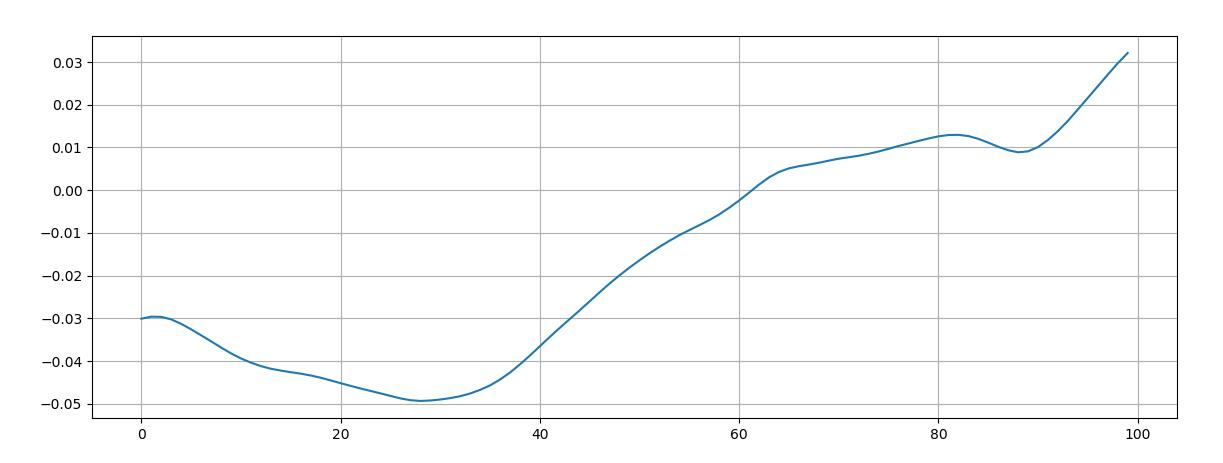zero_crossings = librosa.zero_crossings(x[n0:n1], pad=False)
print(sum(zero_crossings))


### 光谱质心 Spectral Centroid

librosa.feature.spectral_centroid 计算信号中每帧的光谱质心：

spectral_centroids = librosa.feature.spectral_centroid(x, sr=sr)
print(spectral_centroids.shape)
# (2647,)
# Computing the time variable for visualization
frames = range(len(spectral_centroids))
t = librosa.frames_to_time(frames)
# Normalising the spectral centroid for visualisation
def normalize(x, axis=0):
return sklearn.preprocessing.minmax_scale(x, axis=axis)
#Plotting the Spectral Centroid along the waveform
librosa.display.waveplot(x, sr=sr, alpha=0.4)
plt.plot(t, normalize(spectral_centroids), color='r')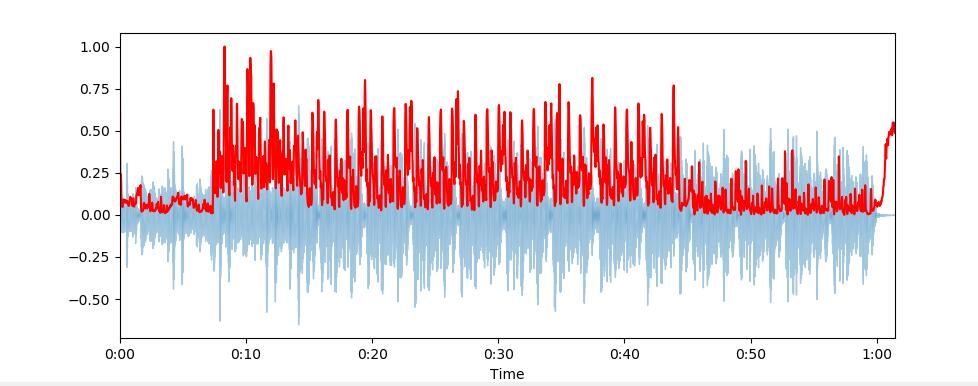### 声谱衰减 Spectral Roll-off

spectral_rolloff = librosa.feature.spectral_rolloff(x+0.01, sr=sr)
librosa.display.waveplot(x, sr=sr, alpha=0.4)
plt.plot(t, normalize(spectral_rolloff), color='r')


### 梅尔频率倒谱系数 MFCC

mfccs = librosa.feature.mfcc(x, sr=fs)
print mfccs.shape
# (20, 97)
#Displaying  the MFCCs:
librosa.display.specshow(mfccs, sr=sr, x_axis='time')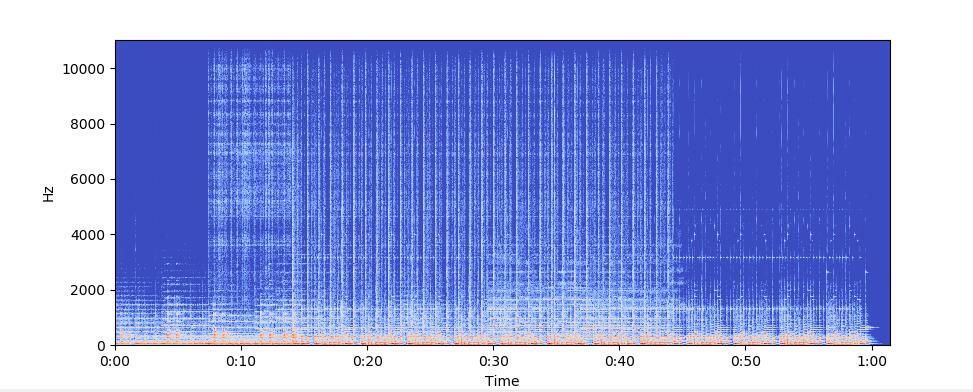import sklearn
mfccs = sklearn.preprocessing.scale(mfccs, axis=1)
print(mfccs.mean(axis=1))
print(mfccs.var(axis=1))
librosa.display.specshow(mfccs, sr=sr, x_axis='time')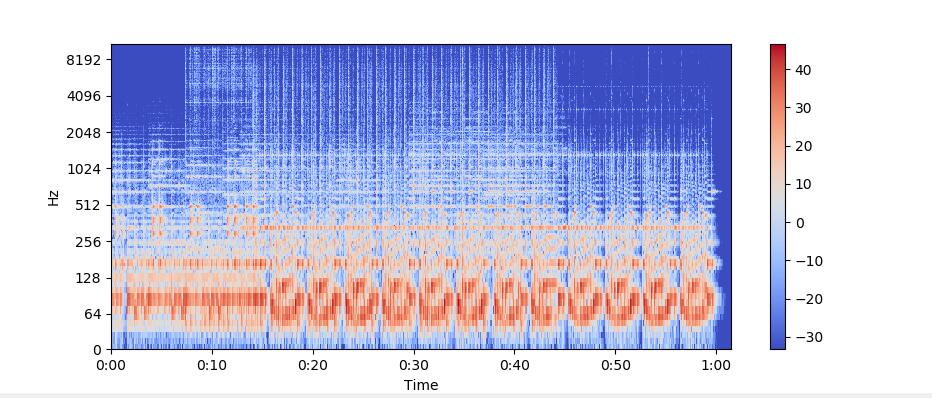### 色度频率 Chroma Frequencies

x, sr = librosa.load('../simple_piano.wav')
hop_length = 512
chromagram = librosa.feature.chroma_stft(x, sr=sr, hop_length=hop_length)
plt.figure(figsize=(15, 5))
librosa.display.specshow(chromagram, x_axis='time', y_axis='chroma', hop_length=hop_length, cmap='coolwarm')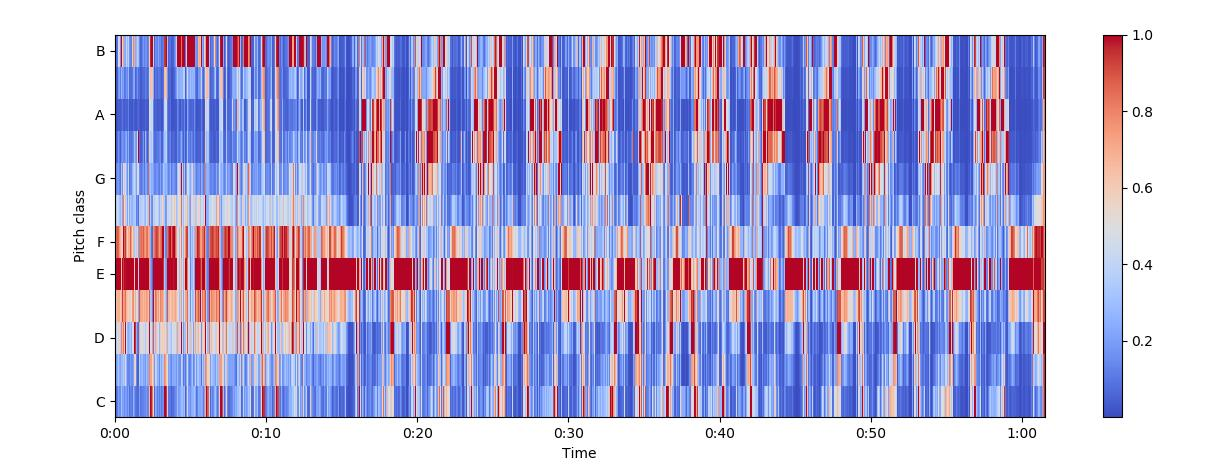## 音频预处理

### Dynamic range compression

def dynamic_range_compression(x, C=1, clip_val=1e-5):
return torch.log(torch.clamp(x, min=clip_val) * C)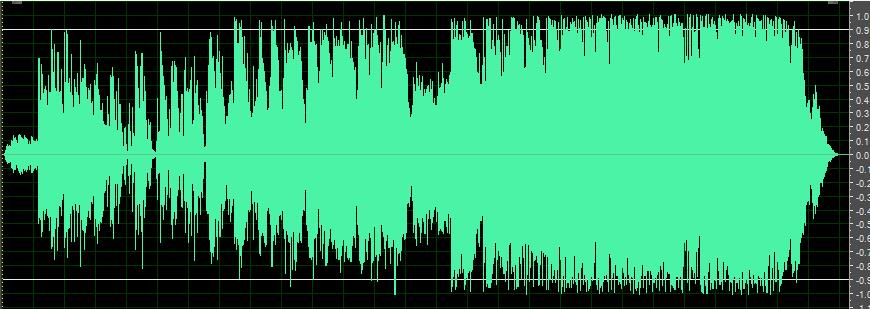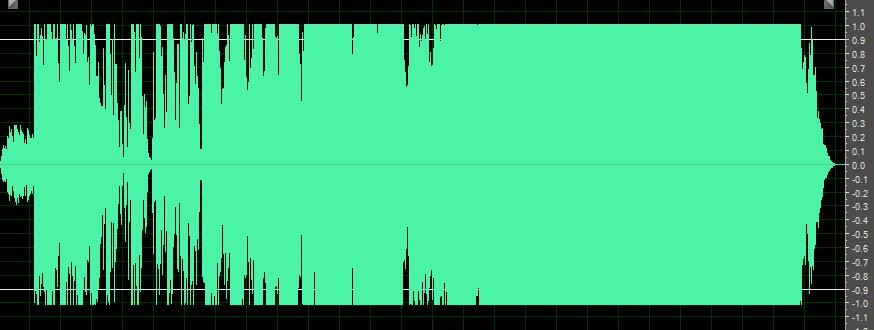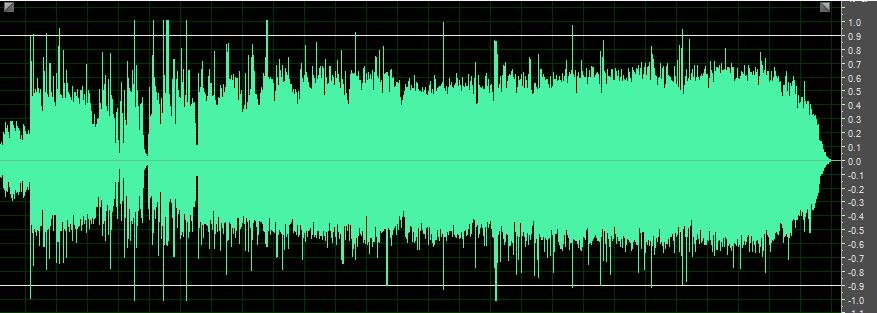## API 附录

### 短时傅里叶变换

librosa.stft(y, n_fft=2048,
hop_length=None,
win_length=None,
window='hann',
center=True,
pad_mode='reflect')


###### 参数：

• y：音频时间序列
• n_fft：FFT窗口大小，n_fft=hop_length+overlapping
• hop_length：帧移，如果未指定，则默认win_length / 4
• win_length：每一帧音频都由window()加窗。窗长win_length，然后用零填充以匹配n_fft
默认win_length=n_fft。
• window：字符串，元组，数字，函数 shape =（n_fft, )
窗口（字符串，元组或数字）
窗函数，例如scipy.signal.hanning
长度为n_fft的向量或数组
• center：bool
如果为True，则填充信号y，以使帧 D [:, t]以y [t * hop_length]为中心
如果为False，则D [:, t]从y [t * hop_length]开始
• dtype：D的复数值类型。默认值为64-bit complex复数
• pad_mode：如果center = True，则在信号的边缘使用填充模式
默认情况下，STFT使用reflection padding

##### 返回：

• STFT矩阵 $shape =（1 + \frac{n-fft}{2}，t）$

### 短时傅里叶逆变换

librosa.istft(stft_matrix,
hop_length=None,
win_length=None,
window='hann',
center=True,
length=None)


#### 参数：

• stft_matrix ：经过STFT之后的矩阵
• hop_length ：帧移，默认为winlength4
• win_length ：窗长，默认为n_fft
• window：字符串，元组，数字，函数或shape = (n_fft, )
窗口（字符串，元组或数字）
窗函数，例如scipy.signal.hanning
长度为n_fft的向量或数组
• center：bool
如果为True，则假定D具有居中的帧
如果False，则假定D具有左对齐的帧
• length：如果提供，则输出y为零填充或剪裁为精确长度音频

• y ：时域信号

### 幅度转dB

librosa.amplitude_to_db(S, ref=1.0)


#### 参数：

• S ：输入幅度
• ref ：参考值，振幅abs（S）相对于ref进行缩放，20∗log10(Sref)

• dB为单位的S

### 功率转dB

librosa.core.power_to_db(S, ref=1.0)


#### 参数：

• S：输入功率
• ref ：参考值，振幅abs(S)相对于ref进行缩放，10∗log10(Sref)

• dB为单位的S

### Mel滤波器组

librosa.filters.mel(sr, n_fft, n_mels=128, fmin=0.0, fmax=None, htk=False, norm=1)


##### 参数：

• sr ：输入信号的采样率
• n_fft ：FFT组件数
• n_mels ：产生的梅尔带数
• fmin ：最低频率（Hz）
• fmax：最高频率（以Hz为单位）。如果为None，则使用fmax = sr / 2.0
• norm：{None，1，np.inf} [标量]
如果为1，则将三角mel权重除以mel带的宽度（区域归一化） 否则，保留所有三角形的峰值为1.0

• Mel变换矩阵

### 提取MFCC系数

MFCC特征是一种在自动语音识别和说话人识别中广泛使用的特征。关于MFCC特征的详细信息，有兴趣的可以参考博客。在librosa中，提取MFCC特征只需要一个函数：

librosa.feature.mfcc(y=None,
sr=22050,
S=None,
n_mfcc=20,
dct_type=2,
norm='ortho',
**kwargs)


#### 参数：

• y：音频数据
• sr：采样率
• S：np.ndarray，对数功能梅尔谱图
• n_mfcc：int>0，要返回的MFCC数量
• dct_type：None, or {1, 2, 3} 离散余弦变换（DCT）类型。默认情况下，使用DCT类型2。
• norm： None or ‘ortho’ 规范。如果dct_type为2或3，则设置norm =’ortho’使用正交DCT基础
标准化不支持dct_type = 1。

• M： MFCC序列